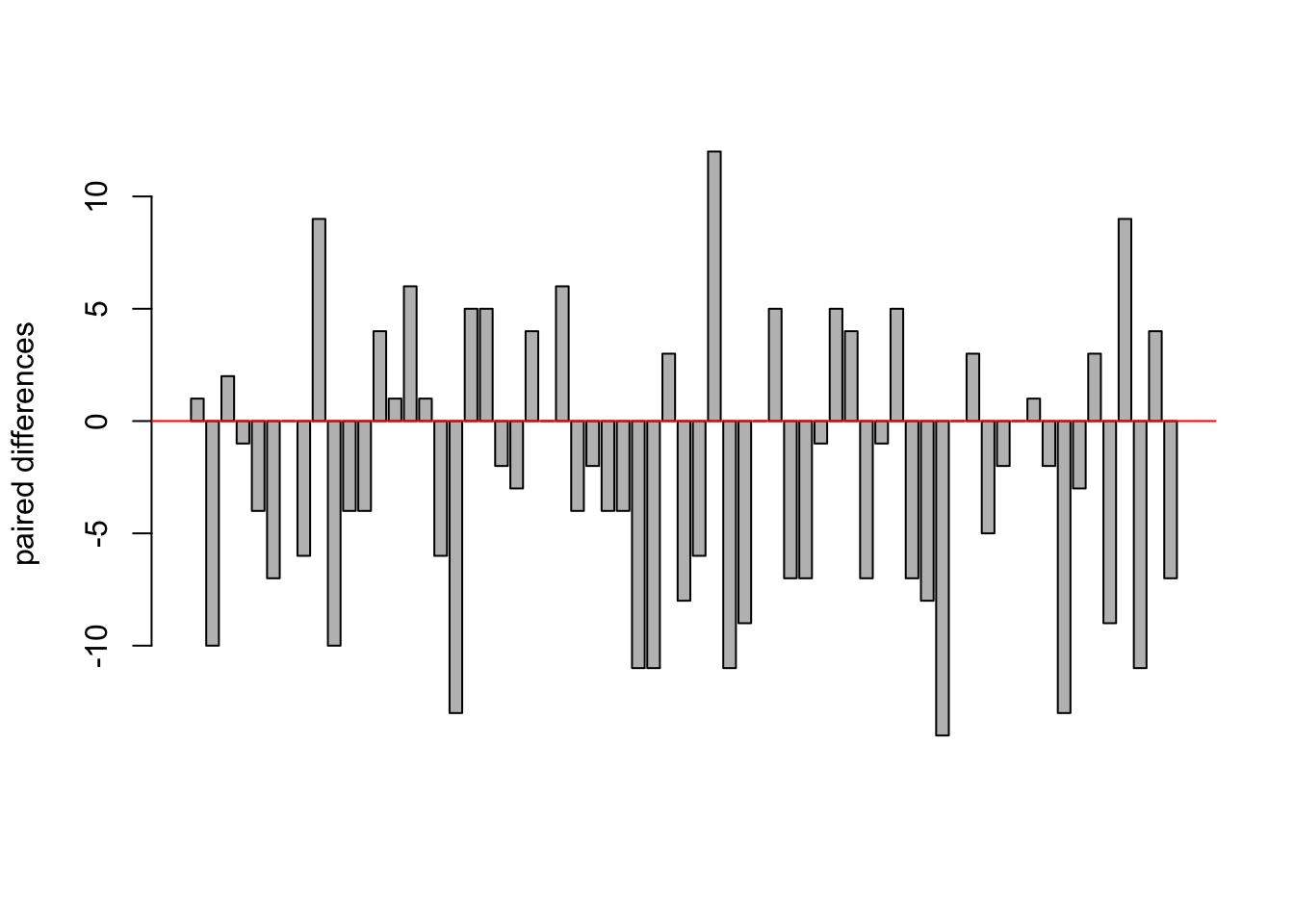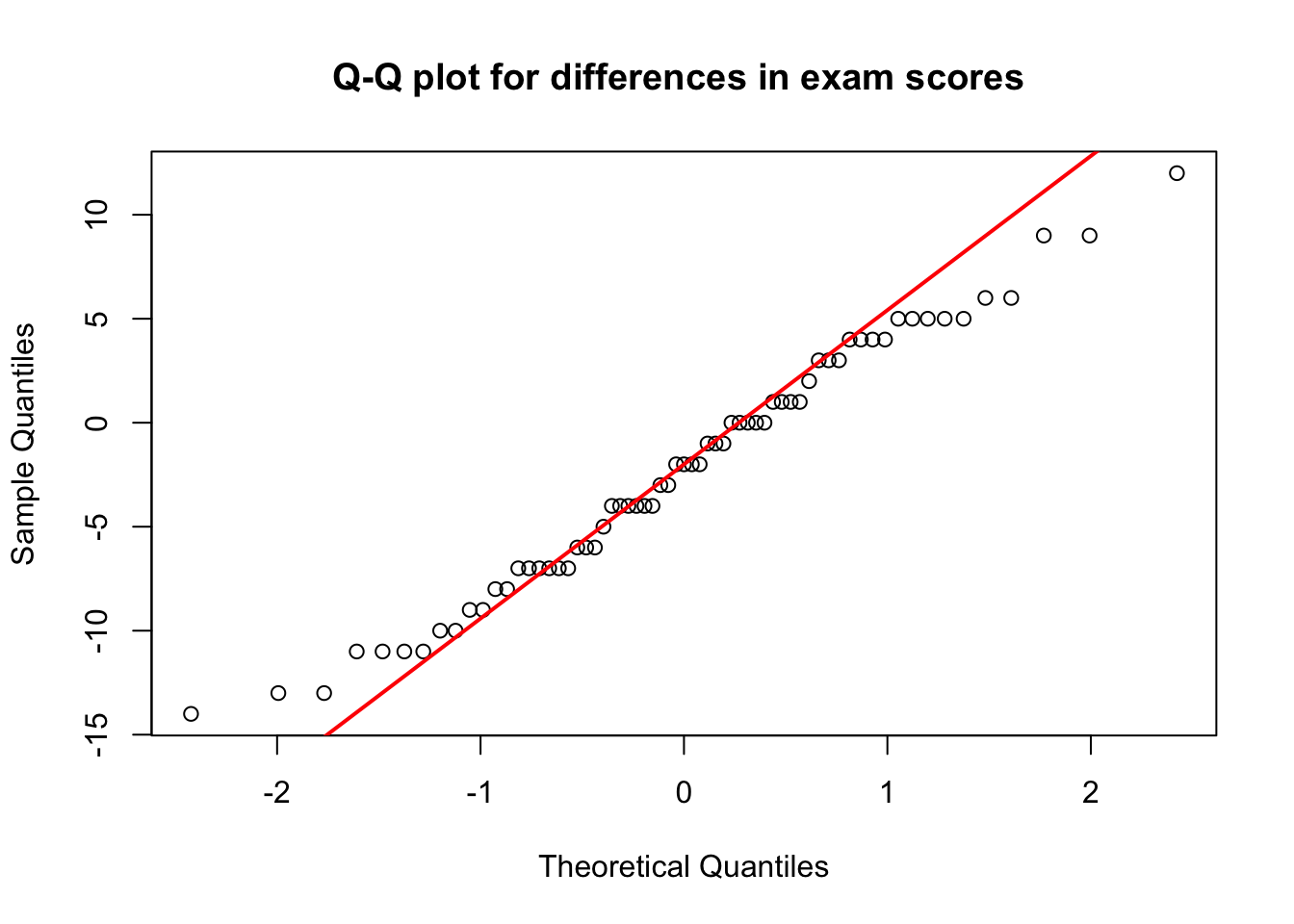Let us now turn to a hypothesis testing procedure for the difference between two population means when the samples are dependent. If for example two data values are collected from the same source (or element), these are called paired or matched samples.

Very often these procedures are applied for Before-After-Control-Impact (BACI) analysis. Imagine a case when you are asked to evaluate the effectiveness of a filtering system in removing air pollutants being released by a factory. In that case one population consists of measurements of air quality before the filtering system is implemented or renewed, and the other population consists of measurements of air quality after the new filter system was installed. In that case you are dealing with paired samples, because the two data sets are collected from the same source, the factory.

In paired samples, the difference between the data values of the two samples is denoted by $$d$$, often called paired difference. Note that the sample size $$n$$ for each sample is equal. The mean of the paired differences for the samples is denoted as $$\bar d$$.

$\bar d = \frac{\sum d}{n}$

The standard deviation of paired differences for two samples, $$s_d$$, is calculated as

$s_d = \sqrt{\frac{\sum d^2 - \frac{(\sum d)^2}{n}}{n-1}}$

Suppose that the paired-difference variable $$d$$ is normally distributed, then the paired $$t$$-statistic is expressed as

$t= \frac{\bar d - (\mu_1-\mu_2)}{\frac{s_d}{\sqrt{n}}}\text{,}$ which simplifies to

$t= \frac{\bar d}{\frac{s_d}{\sqrt{n}}}\text{,}$

if $$\mu_1-\mu_2 = 0$$. The test statistic $$t$$ for paired samples follows a t-distribution with $$df = n - 1$$.

#### Interval Estimation of $$\mu_d$$

The $$100(1-\alpha)$$% confidence interval for $$\mu_d$$ is

$\bar d \pm t \times \frac{s_d}{\sqrt{n}}$ where the value of $$t$$ is obtained from the t-distribution for the given confidence level and $$n-1$$ degrees of freedom.

#### The paired t-test: An example

In order to practice the paired t-test we load the students data set. You may download the students.csv file here. Import the data set and assign a proper name to it.

students <- read.csv("https://userpage.fu-berlin.de/soga/200/2010_data_sets/students.csv")

The students data set consists of 8239 rows, each of them representing a particular student, and 16 columns, each of them corresponding to a variable/feature related to that particular student. These self-explaining variables are: stud.id, name, gender, age, height, weight, religion, nc.score, semester, major, minor, score1, score2, online.tutorial, graduated, salary.

In order to showcase the paired t-test for depended samples we are interested in the question if an online statistics learning tutorial helps students to improve their grades. There are three variables of interest in the students data set. The variable online.tutorial, is a binary variable, which is $$1$$ if the student completed the online statistics learning tutorial or $$0$$ otherwise. The variable score1 and score2 show the grades (0-100) for two exams on mathematics and statistics. The higher the value the better the particular student performed. Please note, that the first exam takes place before the students attended the online statistics learning tutorial. The participation in the online statistics learning tutorial is not mandatory, however the two exams are obligatory for all students. The first exam (score1) takes place at the beginning of the 3rd semester, the second exam (score2) takes place at the end of the 3rd semester.

Basically, there are two research questions of interest. First, we want to examine if the group of students which took the online statistics learning tutorial performs better on the second exam compared to the first exams. Second, we test how the group of students that did not join the online statistics learning tutorial performed on both test.

#### Data preparation

We start with the first research question and focus on those students that took the online statistics learning tutorial.

For data preparation we subset the data set based on the variable online.tutorial, which indicates if the student took the tutorial or not ($$1=\text{yes}, \,0=\text{no}$$). Then we randomly sample 65 students from the data set and extract the two variables of interest, score1 and score2. We store each of them in vector, named score1.sample and score2.sample.

tutorial = subset(students, online.tutorial==1)

n <- 65
random.index <- sample(1:nrow(tutorial), size = n)

score1.sample <- tutorial$score1[random.index] score2.sample <- tutorial$score2[random.index]

Now we compute the paired differences, $$d$$, and plot them.

d <- score1.sample - score2.sample
#plot
barplot(d, ylab = 'paired differences')
abline(h = 0, col = 'red')The plot looks as expected. Some students perform better in the first exam compared the the second exam and vice versa.

In order to check the normality assumption we again rely on a visual inspection of a Q-Q plot. If the variable is normally distributed, the Q-Q plot should be roughly linear. In R we apply the qqnorm() and the qqline() functions for plotting Q-Q plots.

qqnorm(d, main = 'Q-Q plot for differences in exam scores ')
qqline(d, col = 2, lwd = 2)Not super exact and a bit noisy, but the data seems to be roughly normal distributed.

We further calculate $$\bar d$$ the mean of the paired differences

$\bar d = \frac{\sum d}{n}\text{,}$

and $$s_d$$, the standard deviation of the paired differences for two samples

$s_d = \sqrt{\frac{\sum d^2 - \frac{(\sum d)^2}{n}}{n-1}}\text{.}$

#paired difference
d.bar <- sum(d)/length(d)

#standard deviation
s.d <- sqrt((sum(d^2)-(sum(d)^2/length(d)))/(n-1))

#### Hypothesis testing

Now we are ready to apply the paired t-test. Recall our first research question: Does the data provide sufficient evidence to conclude that the mean exam results improve if students take an online statistics learning tutorial?

We follow the step-wise implementation procedure for hypothesis testing.

Step 1: State the null hypothesis $$H_0$$ and alternative hypothesis $$H_A$$

The null hypothesis states that there is no difference in the mean of the exam grades of one exam compared to the other.

$H_0: \quad \mu_1 = \mu_2$

Recall, that the formulation of the alternative hypothesis dictates if we apply a two-sided, a left tailed or a right tailed hypothesis test.

Alternative hypothesis $H_A: \quad \mu_1 < \mu_2$ This formulation results in a left tailed hypothesis test and states that the students on average perform better on the second exam.

Step 2: Decide on the significance level, $$\alpha$$

$\alpha = 0.05$

alpha <- 0.05

Step 3 and 4: Compute the value of the test statistic and the p-value.

For illustration purposes we manually compute the test statistic in R. Recall the equation form above:

$t= \frac{\bar d - (\mu_1-\mu_2)}{\frac{s_d}{\sqrt{n}}}$

If $$H_0$$ is true, then $$\mu_1-\mu_2 = 0$$ and thus, the equation simplifies to

$t= \frac{\bar d}{\frac{s_d}{\sqrt{n}}}\text{.}$

# Compute the value of the test statistic

#paired difference
d.bar <- sum(d)/length(d)

#standard deviation
s.d <- sqrt((sum(d^2)-(sum(d)^2/length(d)))/(n-1))

#test statistic
t <- d.bar/(s.d/sqrt(length(d)))
t
##  -2.993553

The numerical value of the test statistic is -2.9935534.

In order to calculate the p-value we apply the pt() function. Recall how to calculate the degrees of freedom.

$df = n - 1= 64$

# Compute the p-value
df = length(d) - 1
p <- pt(t, df = df, lower.tail = TRUE)
p
##  0.0019579

Step 5: If $$p \le \alpha$$, reject $$H_0$$; otherwise, do not reject $$H_0$$.

p <= alpha
##  TRUE

The p-value is less than the specified significance level of 0.05; we reject $$H_0$$. The test results are statistically significant at the 5% level and provide very strong evidence against the null hypothesis.

Step 6: Interpret the result of the hypothesis test.

$$p = 0.0019579$$. At the 5% significance level, the data provides very strong evidence to conclude that the exam grades of students improve after taking an online statistics learning tutorial.

#### Hypothesis testing in R

We just manually completed a paired t-test in R. That is fine, but now we make use of the full power of the R machinery to obtain the same result as above by just one line of code!

In order to conduct a paired t-test in R we apply the t.test() function. We provide two vectors as data input, and further, we set paired = TRUE, in order to explicitly state, that we apply the paired version of the t-test, and further, we set the alternative argument to alternative = 'less', in order to reflect $$H_A: \; \mu_1 <\mu_2$$.

t.test(x = score1.sample, y = score2.sample, paired = TRUE, alternative = 'less')
##
##  Paired t-test
##
## data:  score1.sample and score2.sample
## t = -2.9936, df = 64, p-value = 0.001958
## alternative hypothesis: true difference in means is less than 0
## 95 percent confidence interval:
##        -Inf -0.9938428
## sample estimates:
## mean of the differences
##               -2.246154

Awesome! Compare the output of the t.test() function with our result from above. They match perfectly! Again, we may conclude that at the 5% significance level, the data provides very strong evidence to conclude that the exam grades of students improve after taking an online statistics learning tutorial.

Before we continue, there is still one research question to be answered. What if there are other reasons for better grades on the second exam? What if the second exam was much easier? What if the students had an awesome lecturer and thus improved during the semester? We test that hypothesis by conducting a paired t-test, explicitly for those students who did not take the online statistics learning tutorial. Now, as we are fully aware of the R machinery we conduct a paired t-test within just a few lines of code.

no.tutorial = subset(students, online.tutorial==0)

n <- 65
random.index <- sample(1:nrow(no.tutorial), size = n)

score1.no.tutorial <- no.tutorial$score1[random.index] score2.no.tutorial <- no.tutorial$score2[random.index]

# conduct paired t-test
t.test(x = score1.no.tutorial, y = score2.no.tutorial, paired = TRUE, alternative = 'less')
##
##  Paired t-test
##
## data:  score1.no.tutorial and score2.no.tutorial
## t = 0.68656, df = 23, p-value = 0.7504
## alternative hypothesis: true difference in means is less than 0
## 95 percent confidence interval:
##      -Inf 2.767928
## sample estimates:
## mean of the differences
##               0.7916667

The p-value is greater than the specified significance level of 0.05; we do not reject $$H_0$$. The test results are statistically significant at the 5% level and provide not sufficient evidence against the null hypothesis.

At the 5% significance level, the data does not provide sufficient evidence to conclude that the exam grades of students, who did not attend the online tutorial, improved.